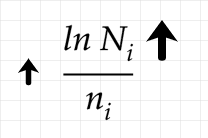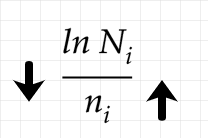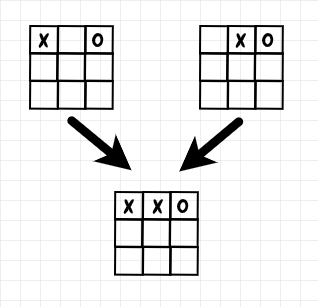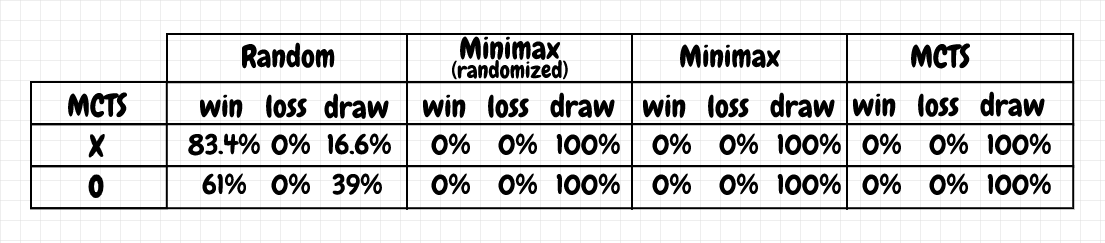07 Aug 2019

# Tic-Tac-Toe with MCTS

### Tic-Tac-Toe (4 Part Series)

So far in this series, we’ve implemented tic-tac-toe with minimax and tabular Q-learning. In this article we’ll use another common technique, MCTS, or Monte Carlo tree search.

Monte Carlo simulations are used in many different areas of computing. Monte Carlo is a heuristic. With a heuristic, we are not guaranteed precisely the correct or the best answer, but we can get an approximation that can often be good enough. Some problems that can’t be solved analytically, or (in a reasonable amount of time) by exhausting all possibilities, become tractable if we run a bunch of simulations instead.

With MCTS, the simulations we perform are called playouts. A playout is a simulation of a single game, often from beginning to end, but sometimes from a given starting point to a given end point. When we get the result of the game, we update the statistics for each game position, or node, that was visited during that playout. The idea is to run a large enough number of playouts to statistically assess the value of taking a given action from a given state. The diagram below shows a part of a single playout for tic-tac-toe:In the diagram, we choose a move for each position until we reach the end of the game (we’ll look at how to make this choice in the next section). In this case, X wins, so we increment the win count and the number of visits for the final position. We increment the win count because X’s previous move that led to this position produced a win for X. For the previous position, we increment the loss count instead of the win count. That’s because O’s move that led to that position ended up as a win for X: For that particular playout, it was a losing move. We go through the move history of the playout, alternating between updating the win and loss count (and the visit count) for each node that was visited. If O wins, then we do the same thing, incrementing the win count for the final position, and then we alternate as before. If it’s a draw, then we just increment the draw count and visit count for each position in the playout. There is a similarity to the way Minimax works here, and in fact, MCTS approximates minimax as we increase the number of simulations.

## Selection and Upper Confidence Bound

How do we select the moves for a given playout? It turns out there are a lot of possible approaches. A simple way to produce a playout would be to choose random moves each time until the end-of-game state, then to update the statistics as described above.

In this article, I’ve chosen to implement UCB-1 (upper confidence bound), a technique that has seen some significant success in machine learning in recent years. This technique of applying an upper confidence bound to MCTS is also sometimes referred to as UCT (upper confidence trees). UCT is an interesting idea used to efficiently select moves during a playout. As with epsilon-greedy (discussed in the previous article), the goal is to find a suitable balance between exploration (trying a move just to see what happens) and exploitation (visiting the node that already has a high value from previous simulations).

To calculate the upper confidence bound, we need the following information:

• Ni: The number of visits to the parent node (the position from which we’re selecting a move)
• ni: The number of visits to a given child node (the position resulting from choosing a move)
• wi: The number of wins for a given child node
• c is a constant that can be adjusted. The default value is the square root of 2, or √2.

Given a position to start from, we apply the following formula for the position resulting from each possible move, then we choose the move with the highest value:The upper confidence bound has two terms. The first is the winrate for a given node. For tic-tac-toe, I use the sum of wins and draws, since we know that a draw is the best possible result if neither player makes a mistake:

``````class Node:
# other code omitted...
def value(self):
if self.visits == 0:
return 0

success_percentage = (self.wins + self.draws) / self.visits
return success_percentage
``````

The second term is the exploration term. This term increases for a given node when it hasn’t been visited very much relative to the number of visits to the parent node. In the numerator, we’ve got the natural log of the number of visits to the parent node. The denominator is the number of visits to the current child node. If we don’t visit a given child node, then the increase in the number of visits to the parent node will gradually increase the exploration term over time. Given enough time, the exploration term will get high enough for that child node to be selected:If we keep increasing the number of visits to the parent node without visiting a given child node, we can see that the overall exploration term above will gradually increase. However, because it is scaled by the natural log, this increase is slow relative to the number of visits.

Each time we do visit a child node, this increments the denominator, which entails a decrease in the exploration term:Since the denominator, unlike the numerator, is not scaled down, if selecting the child node doesn’t increase the winrate, then it will decrease the value of exploring that choice quite rapidly. Overall, if exploring a node has not been promising in the past, it may take a long time before that node is selected again, but it will happen eventually, assuming we run enough playouts.

This approach to the problem of exploration vs exploitation was derived from Hoeffding’s inequality. For more details, see the paper Using Confidence Bounds for Exploitation-Exploration Trade-offs, by Peter Auer.

## Implementation

My implementation of MCTS for tic-tac-toe reuses the `BoardCache` class from the previous articles in this series. This object stores symmetrical board positions as a single key. In order to be able to take advantage of this caching, I had to make some small adjustments to my MCTS implementation. In particular, several distinct positions can lead to the same child position, for instance:To handle this scenario, for a given child node, I keep track of all of its distinct parent nodes: I use the sum of the visits to the parent nodes to compute Ni. Below is the core logic that the MCTS tic-tac-toe player uses to choose a move during a playout:

``````def calculate_value(node_cache, parent_board, board):
node = find_or_create_node(node_cache, board)
if node.visits == 0:
return math.inf

parent_node_visits = node.get_total_visits_for_parent_nodes()

exploration_term = (math.sqrt(2.0)
* math.sqrt(math.log(parent_node_visits) / node.visits))

value = node.value() + exploration_term

return value
``````

If a given child node has not been visited for a playout yet, we make its value infinite. That way, from a given parent node, we give the highest priority to checking every possible child node at least once. Otherwise, we add its current success percentage to the exploration term. Note that once we’ve performed our playouts, we select the actual move to play in a game by just using the highest success rate from the available options.

## Results

From what I understand, UCT should converge to produce the same results as minimax as the number of playouts increases. For tic-tac-toe, I found that pre-training with 4,000 playouts produced results that were close to minimax, and in which the MCTS agent didn’t lose any games (based on 1,000 games played):In practice, I’ve found that MCTS is often used in an “online” mode. That is, instead of pre-training ahead of time, MCTS is implemented live. For example, the original version of AlphaGo used deep learning for move selection and position evaluation, but it also performed MCTS playouts before each move of a real (i.e. non-training) game. It used a combination of the neural network outputs and the results from playouts to decide which move to make. For tic-tac-toe, I tried doing online playouts after each move (without pre-training) and obtained good results (comparable to the table above) with 200 simulations per move.

## Code

The code for this project is available on github (mcts.py):

##nestedsoftware / tictac

### Experimenting with different techniques for playing tic-tac-toe

Demo project for different approaches for playing tic-tac-toe.

Code requires python 3, numpy, and pytest. For the neural network/dqn implementation (qneural.py), pytorch is required.

Install using pipenv:

• `pipenv shell`
• `pipenv install --dev`

Make sure to set `PYTHONPATH` to main project directory:

• In windows, run `path.bat`
• In bash run `source path.sh`

Run tests and demo:

• Run tests: `pytest`
• Run demo: `python -m tictac.main`
• Run neural net demo: `python -m tictac.main_qneural`

Below are the most recent demo results. The current qtable agent plays near-perfect games as O against itself, minimax, and random. Getting good result for the X player was pretty straightforward, but for O it took quite a bit of fiddling with the hyperparameters.

Latest results:

``````C:\Dev\python\tictac>python -m tictac.main
Playing random vs random:
-------------------------
x wins: 60.10%
o wins: 28.90%
draw  : 11.00%

Playing minimax not random vs minimax random:
---------------------------------------------
x wins: 0.00%
o wins: 0.00%
draw  : 100.00%

Playing minimax random vs minimax not random:
---------------------------------------------
x wins: 0.00%
o wins: 0.00%
draw  : 100.00%

Playing minimax not random vs minimax not random:
-------------------------------------------------
x wins: 0.00%
o wins: 0.00%
draw  : 100.00%

Playing minimax random vs minimax random:
-----------------------------------------
x wins: 0.00%
o wins: 0.00%
draw  : 100.00%

Playing minimax random vs random:
---------------------------------
x wins: 96.80%
o wins: 0.00%
draw  : 3.20%

Playing random vs minimax random:
---------------------------------
x wins: 0.00%
o wins: 78.10%
draw  : 21.90%

Training qtable X vs. random...
700/7000 games, using epsilon=0.6...
1400/7000 games, using epsilon=0.5...
2100/7000 games, using epsilon=0.4...
2800/7000 games, using epsilon=0.30000000000000004...
3500/7000 games, using epsilon=0.20000000000000004...
4200/7000 games, using epsilon=0.10000000000000003...
4900/7000 games, using epsilon=2.7755575615628914e-17...
5600/7000 games, using epsilon=0...
6300/7000 games, using epsilon=0...
7000/7000 games, using epsilon=0...
Training qtable O vs. random...
700/7000 games, using epsilon=0.6...
1400/7000 games, using epsilon=0.5...
2100/7000 games, using epsilon=0.4...
2800/7000 games, using epsilon=0.30000000000000004...
3500/7000 games, using epsilon=0.20000000000000004...
4200/7000 games, using epsilon=0.10000000000000003...
4900/7000 games, using epsilon=2.7755575615628914e-17...
5600/7000 games, using epsilon=0...
6300/7000 games, using epsilon=0...
7000/7000 games, using epsilon=0...

Playing qtable vs random:
-------------------------
x wins: 93.10%
o wins: 0.00%
draw  : 6.90%

Playing qtable vs minimax random:
---------------------------------
x wins: 0.00%
o wins: 0.00%
draw  : 100.00%

Playing qtable vs minimax:
--------------------------
x wins: 0.00%
o wins: 0.00%
draw  : 100.00%

Playing random vs qtable:
-------------------------
x wins: 0.00%
o wins: 61.00%
draw  : 39.00%

Playing minimax random vs qtable:
---------------------------------
x wins: 0.00%
o wins: 0.00%
draw  : 100.00%

Playing minimax vs qtable:
--------------------------
x wins: 0.00%
o wins: 0.00%
draw  : 100.00%

Playing qtable vs qtable:
-------------------------
x wins: 0.00%
o wins: 0.00%
draw  : 100.00%

number of items in qtable = 747

Training MCTS...
400/4000 playouts...
800/4000 playouts...
1200/4000 playouts...
1600/4000 playouts...
2000/4000 playouts...
2400/4000 playouts...
2800/4000 playouts...
3200/4000 playouts...
3600/4000 playouts...
4000/4000 playouts...

Playing random vs MCTS:
-----------------------
x wins: 0.00%
o wins: 63.50%
draw  : 36.50%

Playing minimax vs MCTS:
------------------------
x wins: 0.00%
o wins: 0.00%
draw  : 100.00%

Playing minimax random vs MCTS:
-------------------------------
x wins: 0.00%
o wins: 0.00%
draw  : 100.00%

Playing MCTS vs random:
-----------------------
x wins: 81.80%
o wins: 0.00%
draw  : 18.20%

Playing MCTS vs minimax:
------------------------
x wins: 0.00%
o wins: 0.00%
draw  : 100.00%

Playing MCTS vs minimax random:
-------------------------------
x wins: 0.00%
o wins: 0.00%
draw  : 100.00%

Playing MCTS vs MCTS:
---------------------
x wins: 0.00%
o wins: 0.00%
draw  : 100.00%
``````
``````C:\Dev\python\tictac>python -m tictac.main_qneural
Training qlearning X vs. random...
200000/2000000 games, using epsilon=0.6...
400000/2000000 games, using epsilon=0.5...
600000/2000000 games, using epsilon=0.4...
800000/2000000 games, using epsilon=0.30000000000000004...
1000000/2000000 games, using epsilon=0.20000000000000004...
1200000/2000000 games, using epsilon=0.10000000000000003...
1400000/2000000 games, using epsilon=2.7755575615628914e-17...
1600000/2000000 games, using epsilon=0...
1800000/2000000 games, using epsilon=0...
2000000/2000000 games, using epsilon=0...
Training qlearning O vs. random...
200000/2000000 games, using epsilon=0.6...
400000/2000000 games, using epsilon=0.5...
600000/2000000 games, using epsilon=0.4...
800000/2000000 games, using epsilon=0.30000000000000004...
1000000/2000000 games, using epsilon=0.20000000000000004...
1200000/2000000 games, using epsilon=0.10000000000000003...
1400000/2000000 games, using epsilon=2.7755575615628914e-17...
1600000/2000000 games, using epsilon=0...
1800000/2000000 games, using epsilon=0...
2000000/2000000 games, using epsilon=0...

Playing qneural vs random:
--------------------------
x wins: 98.40%
o wins: 0.00%
draw  : 1.60%

Playing qneural vs minimax random:
----------------------------------
x wins: 0.00%
o wins: 0.00%
draw  : 100.00%

Playing qneural vs minimax:
---------------------------
x wins: 0.00%
o wins: 0.00%
draw  : 100.00%

Playing random vs qneural:
--------------------------
x wins: 0.00%
o wins: 75.40%
draw  : 24.60%

Playing minimax random vs qneural:
----------------------------------
x wins: 0.00%
o wins: 0.00%
draw  : 100.00%

Playing minimax vs qneural:
---------------------------
x wins: 0.00%
o wins: 0.00%
draw  : 100.00%

Playing qneural vs qneural:
---------------------------
x wins: 0.00%
o wins: 0.00%
draw  : 100.00%
``````